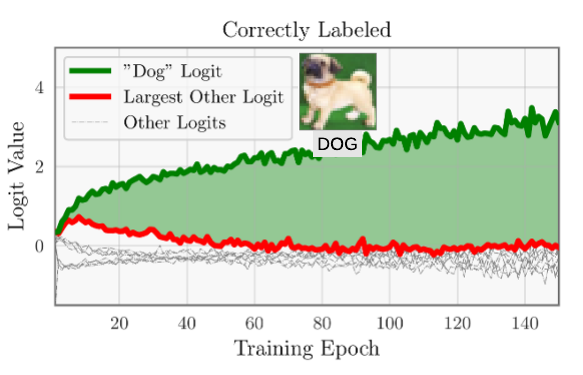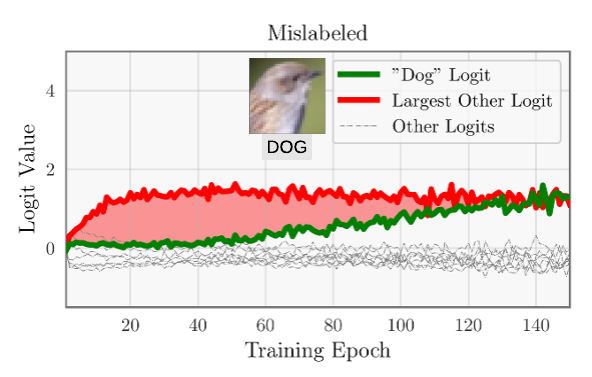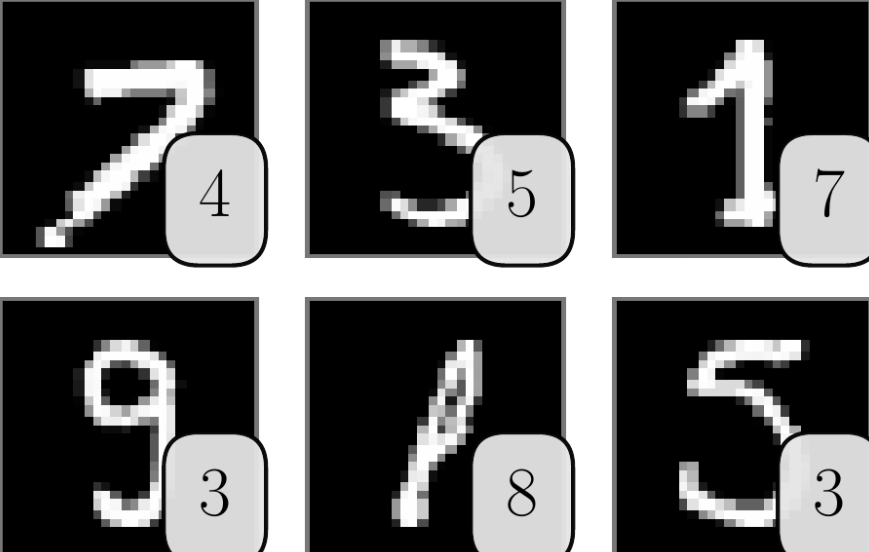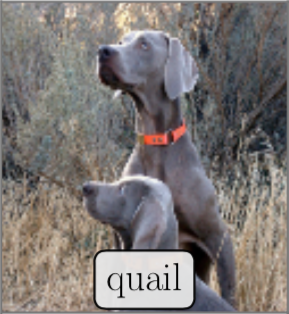# The Area Under the Margin (AUM) StatisticLogits for a correctly-labeled CIFAR10 example. The "dog" logit grows much faster than all others, resulting in a large positive margin and AUM (green area).Logits for a mislabeled CIFAR10 example. The "dog" logit grows slower than the (unobserved) true class logit, resulting in a negative margin and AUM (red area).

## Motivation

Even the most high-power neural network architectures will be prone to error if trained on mislabeled or highly-ambiguous data. Some datasets—especially those that are "weakly-labeled" or annotated by vendor services—are succeptible to such examples. Even some of the most commonly-used datasets, like MNIST and ImageNet, contain several mislabeled examples:Mislabeled training examples from MNIST and ImageNet. These examples were automatically identified using the AUM statistic.

Modern neural networks have sufficient capacity to memorize these mislabeled examples. This memorization will hurt generalization, and thus is a bottleneck limiting the performance of these models. To combat this, we wish to identify and remove samples that are mislabeled, highly-ambigouous, or otherwise harmful to generalization.

## The Area Under the Margin (AUM) Statistic

Mislabeled samples hurt network generalization, while clean samples help generalization. One simple metric that has been shown to be strongly correlated with neural network generalization is the margin. At epoch ..., the margin of sample ... is defined as:

...
... is the model's logit—pre-softmax output—corresponding to class ....)

Intuitively, the margin for any given sample is affected by two forces.

If a sample is correctly labeled, these two forces amplify one another to improve the margin. However, these forces are opposed for mislabeled samples. The first force increases the (incorrect) assigned logit while the second force increases the (hidden) ground-truth logit.

We therefore expect that—on average—a mislabeled sample has a lower margin than a correctly-labeled sample. We capture this by averaging the margin for a given sample over all ... training epochs:

...

This statistic is referred to as the Area Under the Margin, or AUM. It is illustrated in plots above, which plots the logits over time for two training samples. The correctly-labeled sample have large margins, corresponding to a large AUM (green region). The mislabeled sample has a negative margin for most of training, corresponding to a very negative AUM (red region).

## Noteworthy Results

### CIFAR100

We identify 13% of training data that might be mislabeled/ambiguous/harmful to generalization. Simply these data improves error (with a ResNet-32) from 33.0% to 31.8%.

### ImageNet

We identify that 2% of ImageNet data is potentially mislabeled. (This low number is expected, due to ImageNet's rigorous annotation process.) Removing these data does not significantly change ResNet-50 error.Example images from ImageNet with large AUM. (See the mislabeled images above for more high-AUM examples.)

### WebVision-50

This dataset is a standard benchmark for "weakly-labeled" learning. We identify and remove 17% of the data, improving accuracy from 21.4% to 19.8% with a ResNet-50 model.

## Code

We offer a simple PyTorch library (written by Josh Shapiro) for computing the AUM statistic:

``````
pip install aum
``````

This module provides a wrapper around standard PyTorch datasets, as well a as a mechanism for recording the AUM statistic from classification logits. It can be incorporated into any PyTorch classififer in ~10 lines of code.

``````
model.train()
inputs, targets, sample_ids = batch
logits = model(inputs)
records = aum_calculator.update(logits, targets, sample_ids)
# ...
``````

## List of Mislabeled Examples in CIFAR

The following are list of highly-suspicious/low-quality samples identified in the CIFAR dataset. It countains the training set index, the assigned-class label, as well as the AUM score.

## FAQ

How do i determine which samples are mislabeled from the AUM statistic?

AUM provides a ranking of all training points (lower = more likely to be mislabeled). To learn an AUM value that separates clean and mislabeled data, we provide a method of threshold samples (described in Section 3 of the paper). Alternatively, if you have access to a clean validation set, you can run a grid search to find the optimal AUM threshold.

## References

Pleiss, G., Zhang, T., Elenberg, E. R., & Weinberger, K. Q. Identifying Mislabeled Data using the Area Under the Margin Ranking. In Neural Information Processing Systems (2020).